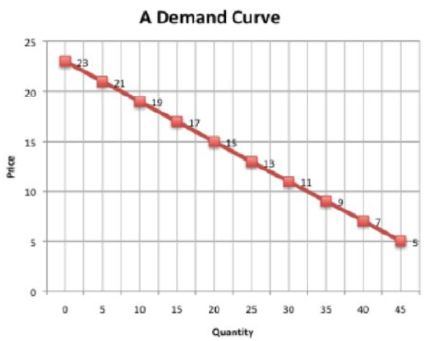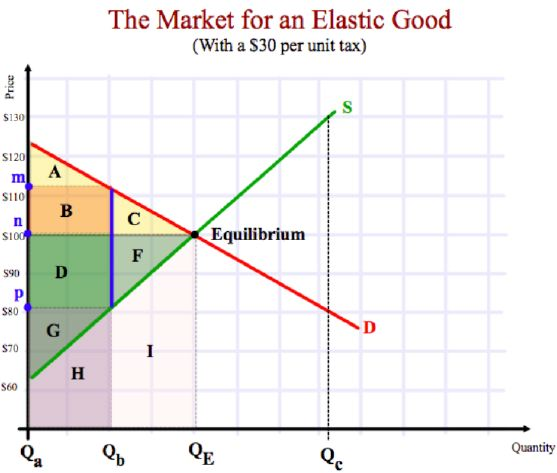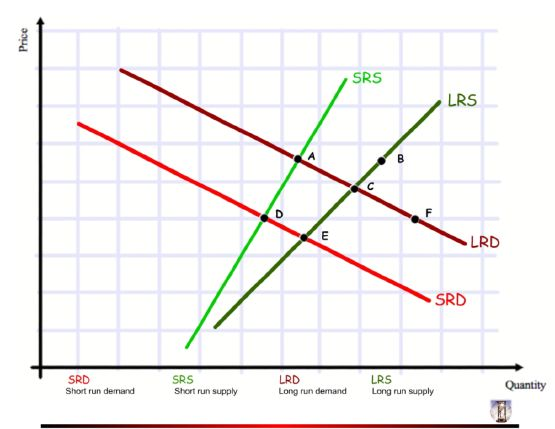Name:    Economics Assessment 02

Multiple Choice
Identify the choice that best completes the statement or answers the question.

1.

Which of the following products is most likely to have an elastic demand?
 a. toothpicks b. insulin c. cigarettes d. automobiles

2.

An increase in the wages of construction workers will:
 a. shift the demand curve for construction workers to the right b. lead to an increase in the quantity supplied of new homes c. shift the supply curve of new homes to the left d. decrease the average annual incomes of construction workers

3.

In which of the following instances does total revenue increase?
 a. price falls and demand has unitary-elasticity b. price falls and supply is inelastic c. price rises and demand is inelastic d. price rises and demand is elastic

4.

Price ceilings and price floors:
 a. make the functioning of free markets more efficient b. alwyas cause shortages c. interfere with the efficient operations of free markets d. shift demand and supply curves

5.

Which statement about quotas is true?
 a. A max. quota greater than equilibrium quantity will create a surplus. b. A max quota set below equilibrium quantity will reduce the amount that a free market would choose to produce, would thus create the incentive for suppliers to break quotas, produce for a black market, and society will pay far more than necessary on legitimate markets, destroying the consumer surplus c. A min. quota set below equilibrium would create shortages and result in black markets for goods and huge losses for suppliers as they must offer artificially low prices in order sell unwanted goods, destroying the producer surplus. d. A min. quota set above equilibrium has no effect.

6.

Which statement about sales taxes and subsidies is true?
 a. Taxes will always raise demand for a product b. Subsidies create surpluses. c. No single side of the market can keep all the benefit of a subsidy or pay all the cost of a tax; it will be shared between consumers and suppliers. d. Taxes and subsidies will always result in inefficient markets that have shortages or surpluses.

7.

The price elasticity of demand indicates:
 a. the extent to which a demand curve shifts as income changes b. the extent to which consumers respond to a change in price c. the length of the demand curve d. how far business executives can stretch their fixed costs

8.

An elastic demand curve is one for which:
 a. the change in price is equal to the change in quantity demanded b. a given percentage change in price causes a smaller percentage change in quantity demanded c. the change in revenue is bigger than the change in quantity demanded d. a given percentage change in price causes a larger percentage change in quantity demanded

9.

Which of the following does not cause the demand for product K to change?
 a. an increase in consumer incomes b. a change in the price of K c. a change in consumer preferences d. a change in the price of substitute product J

10.

Which of the following affects the steepness of the slope of a demand curve?
 a. costs b. tastes and preferences c. income levels among consumers d. customes and cultural values

11.

The price elasticity of supply measures how:
 a. responsive the quantity supplied of X is to changes in the price of X b. responsive quantity supplied is to a change in incomes c. responsive the quantity supplied of Y is to changes in the price of X d. easily labour and capital can be substituted for one another in the production process

12.

Price floors and price ceilings:
 a. both cause surpluses b. both cause shortages c. Price floors cause surpluses and price ceilings cause shortages d. cause the supply and demand curves to shift until equilibrium is established13.

Looking at the demand curve above, at Price = \$15, what is the elasticity coefficient for a price increase of \$2?
 a. 1.875 b. -2/5 c. -0.533 d. -1.875 e. -0.353 f. -2.833 g. The answer is not on this list

14.

If the elasticity of demand is -3, this means
 a. Demand is falling b. The demand curve is elastic at this particular point c. Demand will rise at three times the rate that price rises d. The demand line is elastic

The following questions deal with this graph below.

NOTE: The questions are less memorized knowledge, and more understanding of graphing and concepts. This requires some Thinking/Inquiry (i.e. analysis) as well.Pop out this graph

15.

What area represents the consumer surplus before the tax?
 a. ABC b. B c. A d. AB e. ABCDFG

16.

What is the producer surplus before the tax?
 a. D b. GDF c. DG d. DF e. GH

17.

What is the price level without any government interference?
 a. M b. A c. N d. P

18.

What price do consumers pay after the tax has been introduced?
 a. Unknown b. P c. N d. M

19.

What price do producers receive after the tax is introduced?
 a. Unknown b. P c. N d. M

20.

If producers tried to pass on all of the \$30 tax to consumers what would be the quantity demanded?
 a. Unknown b. Qa c. Qb d. QE e. Qc

21.

If producers were able to pass on all of the tax to consumers, what would be the quantity supplied?
 a. Unknown b. Qa c. Qb d. QE e. Qc

22.

What area represents the amount of tax collected by the government?
 a. BDGH b. N times Qb c. BD d. GH e. DFGHI

23.

What is the revenue received by just the industry (not the government) after the tax is imposed?
 a. GDFHI b. DGH c. GHI d. GH

24.

What area represents how much less revenue the industry receives now that there’s a tax on this product?
 a. ABC b. CFI c. DFI d. BD e. DF

The next questions refer to the graphical illustration below. It represents these two events:

(a) Suppliers are not able to increase their maximum output. However, as time passes, producers become successful in expanding capcity, as well as in building warehousing facilities and improving distribution networks.

(b) Income levels are rising and this developing country’s preference for wearing western-style jeans is rising.Pop out this graph.

(Need help? Try here.)

25.

Which of the following is the first shock?
 a. Prices are raised as sellers witness more demand for their product. b. Quantity supplied rises as sellers try to produce more product since the price went up. c. (i) Preferences and (ii) wealth shift the demand curve to the right because consumers (i) want more and (ii) can afford more at all price levels. d. (i) Increased output and more producers shift the supply curve, and (i) better distribution and warehousing change time to market and increase suppliers responsiveness (i.e. lowers slope/increases elasticity).

26.

Which of the following is the second shock?
 a. Prices are raised as sellers witness more demand for their product. b. Quantity supplied rises as sellers try to produce more product since the price went up. c. (i) Preferences and (ii) wealth shift the demand curve to the right because consumers (i) want more and (ii) can afford more at all price levels. d. (i) Increased output and more producers shift the supply curve, and (i) better distribution and warehousing change time to market and increase suppliers responsiveness (i.e. lowers slope/increases elasticity).

27.

What will the end result (in the long-run) of all of the forces and market shocks described in the graph?
 a. The equilibrium Quantity rises, and the market Price falls b. The equilibrium Quantity falls, and the market Price rises c. The equilibrium Quantity rises, and the market Price rises d. The equilibrium Quantity falls, and the market Price falls

28.

What letter corresponds to the initial equilibrium of the jean market in this developing economy?
 a. A b. B c. C d. D e. E f. F g. Not shown

29.

Which letter corresponds to the price/quantity demanded point of the jean market after its first “shock”?
 a. A b. B c. C d. D e. E f. F

30.

Which letter corresponds to the price/quantity supplied point of the jean market after its first “shock”?
 a. A b. B c. C d. D e. E f. F

31.

If a new equilibrium is reached before the second shock, where would it be?
 a. A b. B c. C d. D e. E f. F

32.

What is the price/quantity demanded point immediately after the second shock?
 a. A b. B c. C d. D e. E f. F

33.

What is the price/quantity supplied point immediately after the second shock?
 a. A b. B c. C d. D e. E f. F

34.

Where is the final equilibrium after all events described have taken place?
 a. A b. B c. C d. D e. E f. F

Matching - NOTE: Not all terms will be used.

 a. Utility k. Price floor b. Surplus l. Price ceiling c. Shortage m. Quota d. Shift in demand curves n. Marginal benefit e. Equilibrium price o. Consumer surplus f. Law of demand and supply p. Substitute good g. Demand curve q. Complementary good h. Quantity supplied r. Law of diminishing marginal utility i. Market demand s. Price elasticity of demand j. Inferior good t. Inelastic demand

35.

The difference between total benefit and total expenditure for a given quantity of a product

36.

The responsiveness of a product's quantity demanded to a change in its price

37.

The legal minimum price set above equilibrium

38.

A good whose increase in price increases demand for another good

39.

The sum of all consumers' quantity demanded for a product at each price

40.

The price at which quantity demanded equals quantity supplied

41.

The number of units that producers want to sell at a given price

42.

The legal maximum price set below equilibrium

43.

The excess of quantity demanded over quantity supplied

44.

The excess of quantity supplied over quantity demanded

45.

The theory that additional units of a particular product generally have a decreasing value to the consumer

46.

A legal limit set by the government on the production of a product

47.

A good whose increase in price decreases demand for another good